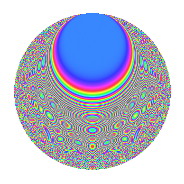# Properties

 Label 1575.2.iLevel 1575 Weight 2 Character orbit i Rep. character $$\chi_{1575}(526,\cdot)$$ Character field $$\Q(\zeta_{3})$$ Dimension 228 Sturm bound 480

# Related objects

## Defining parameters

 Level: $$N$$ = $$1575 = 3^{2} \cdot 5^{2} \cdot 7$$ Weight: $$k$$ = $$2$$ Character orbit: $$[\chi]$$ = 1575.i (of order $$3$$ and degree $$2$$) Character conductor: $$\operatorname{cond}(\chi)$$ = $$9$$ Character field: $$\Q(\zeta_{3})$$ Sturm bound: $$480$$

## Dimensions

The following table gives the dimensions of various subspaces of $$M_{2}(1575, [\chi])$$.

Total New Old
Modular forms 504 228 276
Cusp forms 456 228 228
Eisenstein series 48 0 48

## Trace form

 $$228q - 2q^{2} - 114q^{4} - 8q^{6} - 8q^{9} + O(q^{10})$$ $$228q - 2q^{2} - 114q^{4} - 8q^{6} - 8q^{9} + 8q^{11} + 32q^{12} + 4q^{14} - 114q^{16} - 12q^{17} - 26q^{18} - 12q^{19} + 2q^{21} + 6q^{22} - 12q^{23} + 48q^{24} + 56q^{26} - 12q^{27} + 22q^{29} - 6q^{31} - 12q^{32} + 24q^{33} + 18q^{34} - 2q^{36} + 12q^{37} + 18q^{38} - 20q^{39} + 6q^{41} - 20q^{42} + 6q^{43} - 100q^{44} - 24q^{46} - 30q^{47} - 14q^{48} - 114q^{49} + 50q^{51} + 18q^{52} + 56q^{53} - 20q^{54} + 12q^{56} + 46q^{57} + 18q^{58} + 52q^{59} + 24q^{62} + 168q^{64} - 130q^{66} + 92q^{68} - 28q^{69} - 84q^{71} + 28q^{72} - 36q^{73} + 4q^{74} + 30q^{76} - 8q^{77} + 8q^{78} - 6q^{79} - 92q^{81} - 96q^{82} - 10q^{83} - 30q^{84} - 8q^{86} + 4q^{87} + 42q^{88} + 100q^{89} + 12q^{91} - 86q^{92} - 30q^{93} + 42q^{94} + 68q^{96} + 36q^{97} + 4q^{98} - 52q^{99} + O(q^{100})$$

## Decomposition of $$S_{2}^{\mathrm{new}}(1575, [\chi])$$ into newform subspaces

The newforms in this space have not yet been added to the LMFDB.

## Decomposition of $$S_{2}^{\mathrm{old}}(1575, [\chi])$$ into lower level spaces

$$S_{2}^{\mathrm{old}}(1575, [\chi]) \cong$$ $$S_{2}^{\mathrm{new}}(45, [\chi])$$$$^{\oplus 4}$$$$\oplus$$$$S_{2}^{\mathrm{new}}(63, [\chi])$$$$^{\oplus 3}$$$$\oplus$$$$S_{2}^{\mathrm{new}}(225, [\chi])$$$$^{\oplus 2}$$$$\oplus$$$$S_{2}^{\mathrm{new}}(315, [\chi])$$$$^{\oplus 2}$$

## Hecke Characteristic Polynomials

There are no characteristic polynomials of Hecke operators in the database